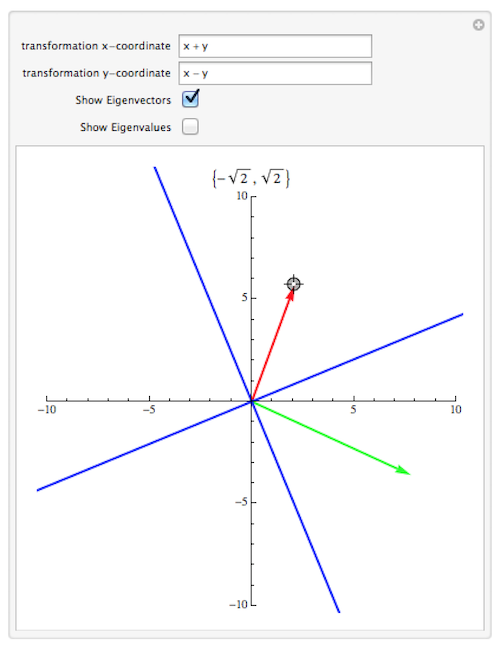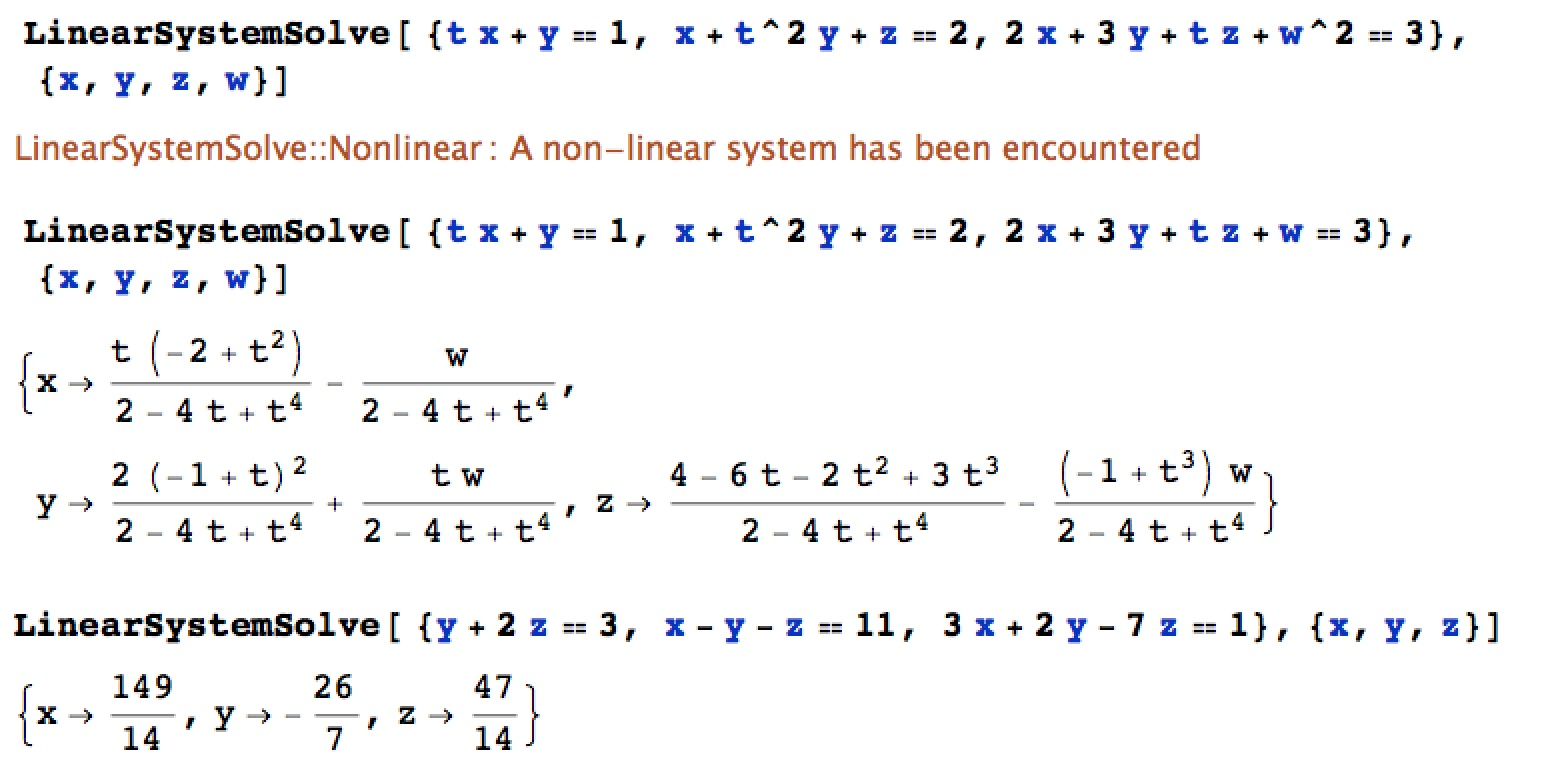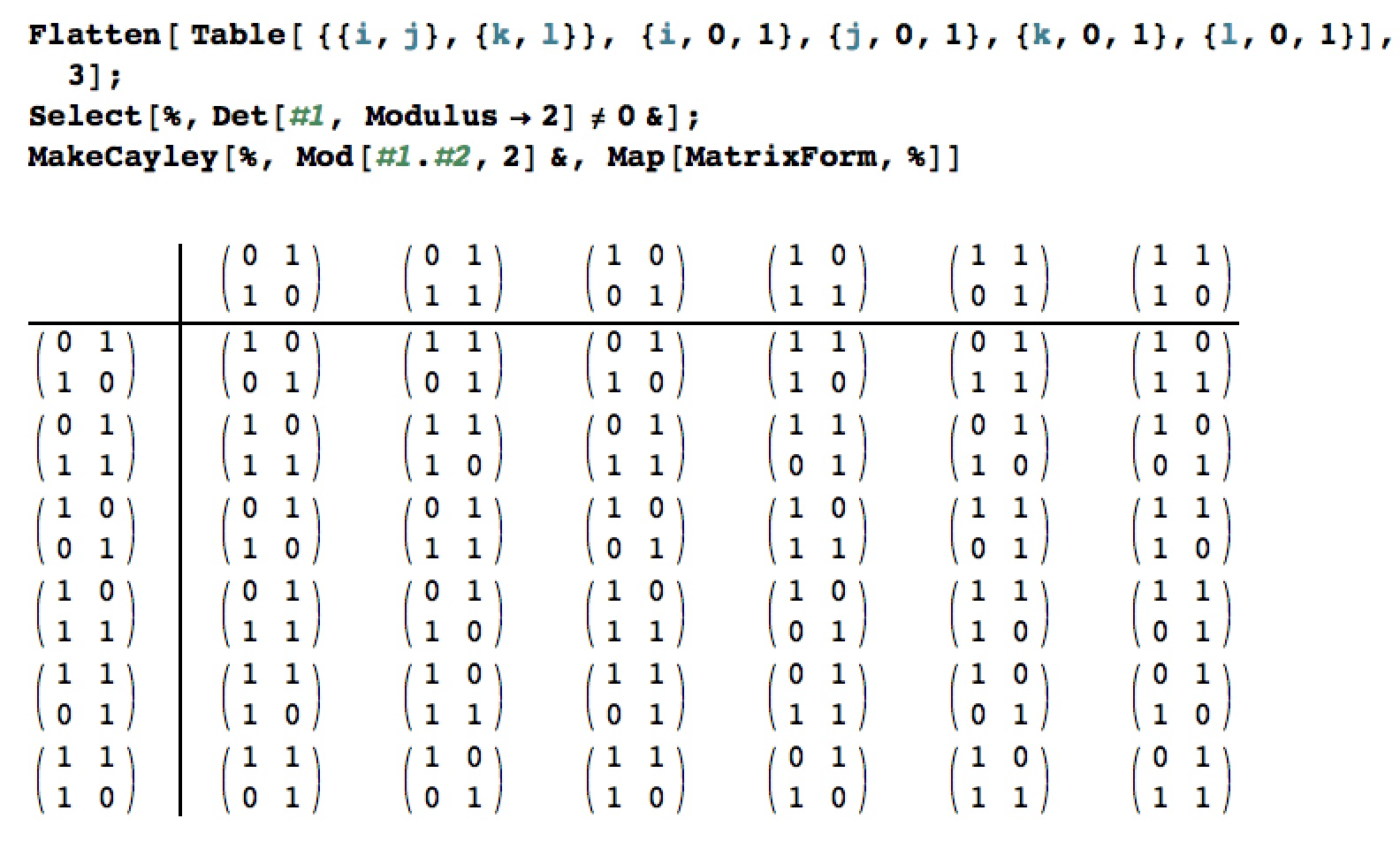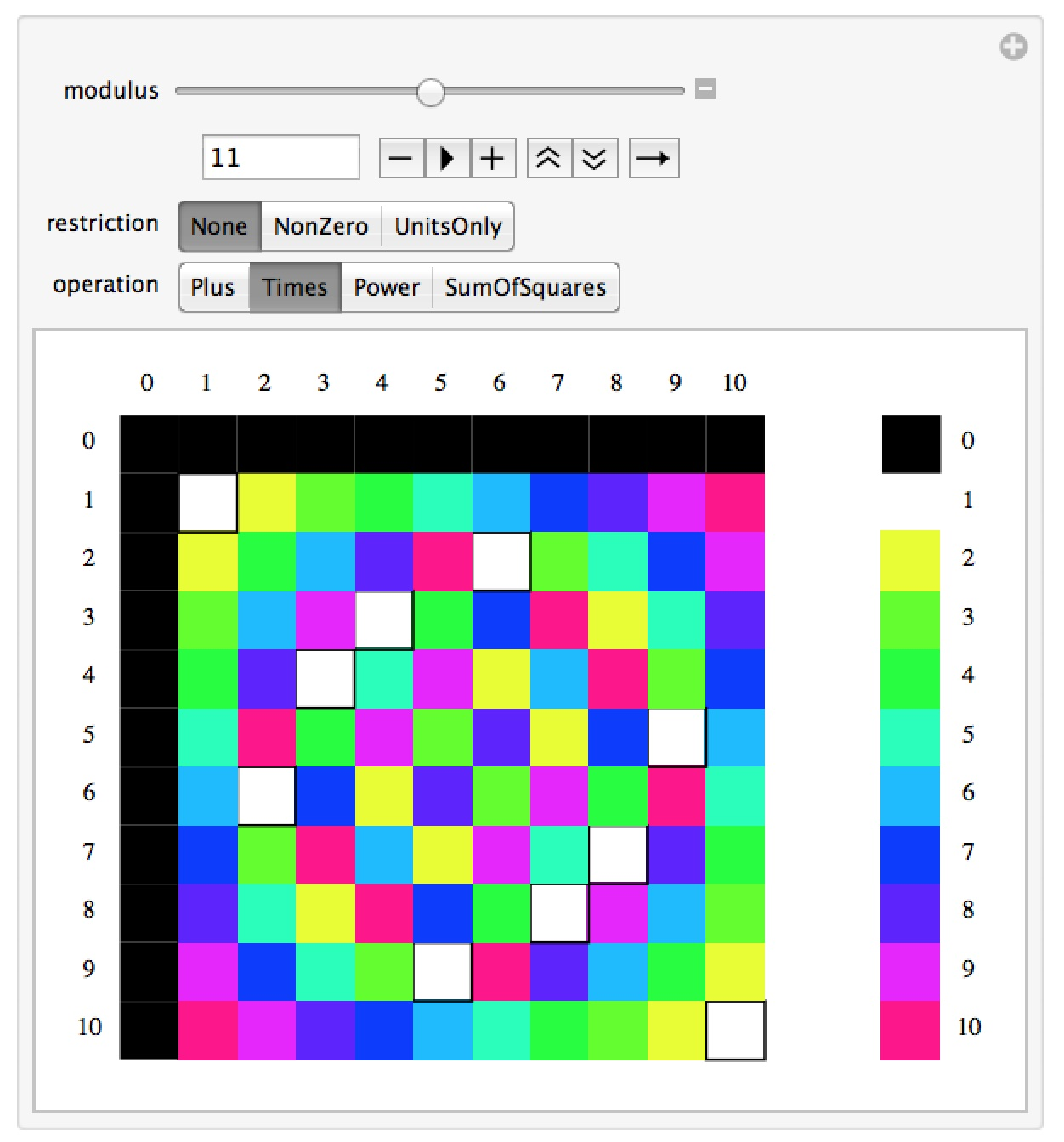# Mathematica Notebooks for Linear & Abstract Algebra

Important Note: The links for the notebooks open a new window or tab with a Google Drive page – the current settings for our homepages won’t allow me to host mathematica notebooks locally.

## Graphical Eigenvectors

This notebook gives a graphical demonstration of what it means to be an eigenvector.  In this case you can enter in a linear transformation in x and y.  You can then drag around the terminal point of a vector (in red) and the computer will plot the image of your vector under the transformation in green.  Your vector will be an eigenvector if the result vector is a scalar multiple of it  – that is, if it is in the same or opposite direction.  Of course you will only be able to do this if the eigenvalues for the linear transformation are real.  You can also choose to have the true eigenvectors or eigenvalues displayed for reference.## A “home-brewed” solver for systems of linear equations

This notebook contains some code we wrote in our Technology 2 class to solve systems of linear equations (essentially duplicating a very small part of the functionality of Mathematica’s Solve command).  The code works by first checking if a system is linear in given variables, then putting the system in matrix form, performing Gauss-Jordan elimination on the resulting matrix, and then converting the reduced matrix to standard Mathematica notation.  Solving a linear system is a great example of both being able to understand and algorithm and then code it.## Cayley Tables

This notebook contains codes to let you quickly create “Cayley Tables” – operation tables that are commonly used to understand small groups and rings.  The commands CayleyTable and MakeRingTables allow you do to tables for either one or two operations respectively.  Both allow for the use of arbitrarily labels for the elements, and the MakeRingTables command requires you to put in labels for each of the tables so you can tell which is which.  The example below shows a quick construction of the Cayley table for the group GL(2,Z_2).  Once you have the Cayley table you can easily see that this is a group of 6 elements, and since the table isn’t symmetric about the main diagonal the group is non-Abelian, and therefore must be isomorphic to S_3.  The table can also be used to computer orders of elements, centralizers, etc.